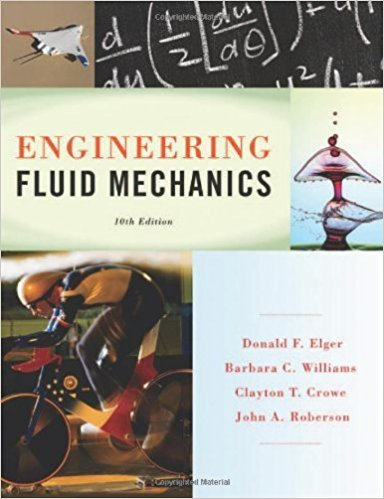×
×

# A laminar flow occurs between two horizontal parallel plates under a pressure gradientISBN: 9781118164297 288

## Solution for problem 2.36 Chapter 2

Engineering Fluid Mechanics | 10th Edition

• Textbook Solutions
• 2901 Step-by-step solutions solved by professors and subject experts
• Get 24/7 help from StudySoup virtual teaching assistantsEngineering Fluid Mechanics | 10th Edition

4 5 1 282 Reviews
31
3
Problem 2.36

A laminar flow occurs between two horizontal parallel plates under a pressure gradient dplds (p decreases in the positives direction). The upper plate moves left (negative) at elocity u,. The expression for local velocity u is given as 1 dp y u = - - (Hy - y2) + u,- 2f-L ds H a. ls the magnitude of the shear stress greater at the moving plate (y = H) or at the stationary plate (y = 0)? b. Derive an expression for they position of zero shear stress. c. Derive an expression for the plate speed u1 required to make the shear stress zero at y = 0.

Step-by-Step Solution:
Step 1 of 3

7/22/2017 OneNote Online Lecture 3/5/14 Wednesday, March 05, 22:30 PM https://onedrive.live.com/view.aspxref=button&Bsrc=SMIT&resid=36773184373A8F0B!1292&cid=36773184373a8f0b&app=OneNote&authkey=Ao_s…...

Step 2 of 3

Step 3 of 3

##### ISBN: 9781118164297

Since the solution to 2.36 from 2 chapter was answered, more than 237 students have viewed the full step-by-step answer. The answer to “A laminar flow occurs between two horizontal parallel plates under a pressure gradient dplds (p decreases in the positives direction). The upper plate moves left (negative) at elocity u,. The expression for local velocity u is given as 1 dp y u = - - (Hy - y2) + u,- 2f-L ds H a. ls the magnitude of the shear stress greater at the moving plate (y = H) or at the stationary plate (y = 0)? b. Derive an expression for they position of zero shear stress. c. Derive an expression for the plate speed u1 required to make the shear stress zero at y = 0.” is broken down into a number of easy to follow steps, and 108 words. Engineering Fluid Mechanics was written by and is associated to the ISBN: 9781118164297. This textbook survival guide was created for the textbook: Engineering Fluid Mechanics, edition: 10. This full solution covers the following key subjects: . This expansive textbook survival guide covers 16 chapters, and 1172 solutions. The full step-by-step solution to problem: 2.36 from chapter: 2 was answered by , our top Physics solution expert on 01/25/18, 04:53PM.

Unlock Textbook Solution